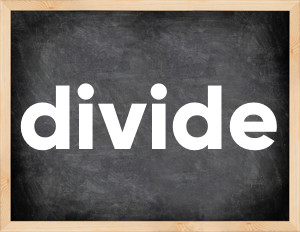# Divide past tenseThe English verb 'divide' is pronounced as [dɪˈvaɪd].
Related to: regular verbs.
3 forms of verb divide: Infinitive (divide), Past Simple - (divided), Past Participle - (divided).

## Here are the past tense forms of the verb divide

👉 Forms of verb divide in future and past simple and past participle.
❓ What is the past tense of divide.

## Divide: Past, Present, and Participle Forms

Base Form Past Simple Past Participle
divide [dɪˈvaɪd]

divided [dɪˈvaɪdɪd]

divided [dɪˈvaɪdɪd]

## What are the 2nd and 3rd forms of the verb divide?

🎓 What are the past simple, future simple, present perfect, past perfect, and future perfect forms of the base form (infinitive) 'divide'?

### Learn the three forms of the English verb 'divide'

• the first form (V1) is 'divide' used in present simple and future simple tenses.
• the second form (V2) is 'divided' used in past simple tense.
• the third form (V3) is 'divided' used in present perfect and past perfect tenses.

## What are the past tense and past participle of divide?

The past tense and past participle of divide are: divide in past simple is divided, and past participle is divided.

### What is the past tense of divide?

The past tense of the verb "divide" is "divided", and the past participle is "divided".

### Verb Tenses

Past simple — divide in past simple divided (V2).
Future simple — divide in future simple is divide (will + V1).
Present Perfect — divide in present perfect tense is divided (have/has + V3).
Past Perfect — divide in past perfect tense is divided (had + V3).

### divide regular or irregular verb?

👉 Is 'divide' a regular or irregular verb? The verb 'divide' is regular verb.

## Examples of Verb divide in Sentences

•   They have so many differences that divide them. (Present Simple)
•   Our camp was divided into 24 parts. (Past Simple)
•   The group has divided into two main parts. (Present Perfect)
•   Mother divided the pie into eight pieces for all of the guests. (Past Simple)
•   Paul divided his salary between his children. (Past Simple)
•   What's 72 divided by 9? (Present Simple)
•   Do you want to build a fence to divide the flower garden from the vegetable garde? (Present Simple)
•   She divides her time between work and faamily. (Present Simple)
•   Why did cells begin to divide out of control? (Past Simple)
•   We reached the spot at which our ways divided, but didn't want to separate. (Past Simple)

Along with divide, words are popular describe and strew.

Verbs by letter: , , , , , , , , , , , , , , , , , , , , , , , , .